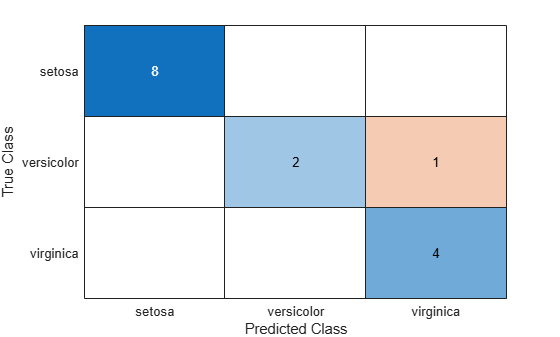# predict

Classify observations using ensemble of classification models

## Syntax

``labels = predict(Mdl,X)``
``labels = predict(Mdl,X,Name,Value)``
``````[labels,scores] = predict(___)``````

## Description

````labels = predict(Mdl,X)` returns a vector of predicted class labels for the predictor data in the table or matrix `X`, based on the full or compact, trained classification ensemble `Mdl`.```
````labels = predict(Mdl,X,Name,Value)` uses additional options specified by one or more `Name,Value` pair arguments.```
``````[labels,scores] = predict(___)``` also returns a matrix of classification scores (`scores`), indicating the likelihood that a label comes from a particular class, using any of the input arguments in the previous syntaxes. For each observation in `X`, the predicted class label corresponds to the maximum score among all classes.```

## Input Arguments

 `Mdl` A classification ensemble created by `fitcensemble` or a compact classification ensemble created by `compact`. `X` Predictor data to be classified, specified as a numeric matrix or table. Each row of `X` corresponds to one observation, and each column corresponds to one variable. For a numeric matrix: The variables making up the columns of `X` must have the same order as the predictor variables that trained `Mdl`.If you trained `Mdl` using a table (for example, `Tbl`), then `X` can be a numeric matrix if `Tbl` contains all numeric predictor variables. To treat numeric predictors in `Tbl` as categorical during training, identify categorical predictors using the `CategoricalPredictors` name-value pair argument of `fitcensemble`. If `Tbl` contains heterogeneous predictor variables (for example, numeric and categorical data types) and `X` is a numeric matrix, then `predict` throws an error. For a table: `predict` does not support multicolumn variables or cell arrays other than cell arrays of character vectors.If you trained `Mdl` using a table (for example, `Tbl`), then all predictor variables in `X` must have the same variable names and be of the same data types as those that trained `Mdl` (stored in `Mdl.PredictorNames`). However, the column order of `X` does not need to correspond to the column order of `Tbl`. `Tbl` and `X` can contain additional variables (response variables, observation weights, etc.), but `predict` ignores them.If you trained `Mdl` using a numeric matrix, then the predictor names in `Mdl.PredictorNames` and corresponding predictor variable names in `X` must be the same. To specify predictor names during training, see the `PredictorNames` name-value pair argument of `fitcensemble`. All predictor variables in `X` must be numeric vectors. `X` can contain additional variables (response variables, observation weights, etc.), but `predict` ignores them.

### Name-Value Arguments

Specify optional pairs of arguments as `Name1=Value1,...,NameN=ValueN`, where `Name` is the argument name and `Value` is the corresponding value. Name-value arguments must appear after other arguments, but the order of the pairs does not matter.

Before R2021a, use commas to separate each name and value, and enclose `Name` in quotes.

 `Learners` Indices of weak learners `predict` uses for computation of responses, a numeric vector. Default: `1:T`, where `T` is the number of weak learners in `Mdl` `UseObsForLearner` A logical matrix of size `N`-by-`T`, where: `N` is the number of rows of `X`.`T` is the number of weak learners in `Mdl`. When `UseObsForLearner(i,j)` is `true`, learner `j` is used in predicting the class of row `i` of `X`. Default: `true(N,T)` `UseParallel` Indication to perform inference in parallel, specified as `false` (compute serially) or `true` (compute in parallel). Parallel computation requires Parallel Computing Toolbox™. Parallel inference can be faster than serial inference, especially for large datasets. Parallel computation is supported only for tree learners.Default: `false`

## Output Arguments

 `labels` Vector of classification labels. `labels` has the same data type as the labels used in training `Mdl`. (The software treats string arrays as cell arrays of character vectors.) `scores` A matrix with one row per observation and one column per class. For each observation and each class, the score represents the confidence that the observation originates from that class. A higher score indicates a higher confidence. For more information, see Score (ensemble).

## Examples

expand all

Load Fisher's iris data set. Determine the sample size.

```load fisheriris N = size(meas,1);```

Partition the data into training and test sets. Hold out 10% of the data for testing.

```rng(1); % For reproducibility cvp = cvpartition(N,'Holdout',0.1); idxTrn = training(cvp); % Training set indices idxTest = test(cvp); % Test set indices```

Store the training data in a table.

```tblTrn = array2table(meas(idxTrn,:)); tblTrn.Y = species(idxTrn);```

Train a classification ensemble using AdaBoostM2 and the training set. Specify tree stumps as the weak learners.

```t = templateTree('MaxNumSplits',1); Mdl = fitcensemble(tblTrn,'Y','Method','AdaBoostM2','Learners',t);```

Predict labels for the test set. You trained model using a table of data, but you can predict labels using a matrix.

`labels = predict(Mdl,meas(idxTest,:));`

Construct a confusion matrix for the test set.

`confusionchart(species(idxTest),labels)``Mdl` misclassifies one versicolor iris as virginica in the test set.

expand all

## Alternative Functionality

To integrate the prediction of an ensemble into Simulink®, you can use the ClassificationEnsemble Predict block in the Statistics and Machine Learning Toolbox™ library or a MATLAB® Function block with the `predict` function. For examples, see Predict Class Labels Using ClassificationEnsemble Predict Block and Predict Class Labels Using MATLAB Function Block.

When deciding which approach to use, consider the following:

• If you use the Statistics and Machine Learning Toolbox library block, you can use the Fixed-Point Tool (Fixed-Point Designer) to convert a floating-point model to fixed point.

• Support for variable-size arrays must be enabled for a MATLAB Function block with the `predict` function.

• If you use a MATLAB Function block, you can use MATLAB functions for preprocessing or post-processing before or after predictions in the same MATLAB Function block.

## Version History

Introduced in R2011a